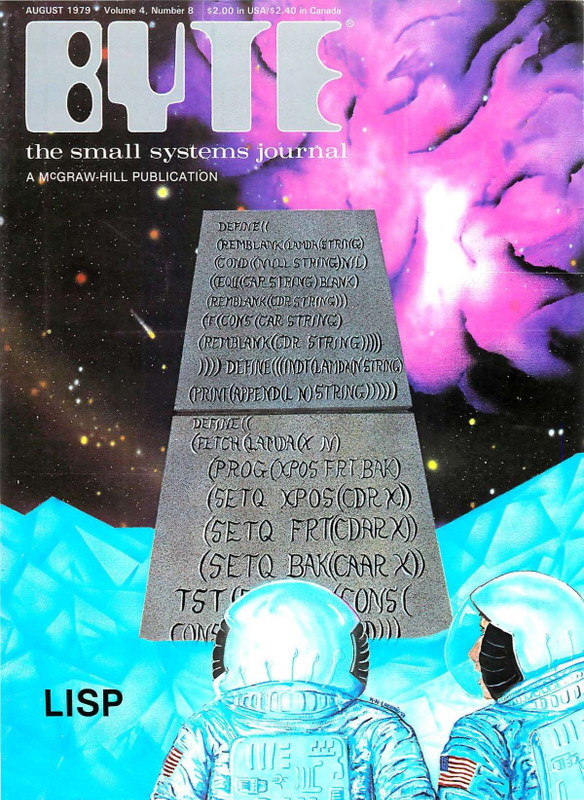The image from this post is from a tweet by Paige Bailey (@DynamicWebPaige). It's from the August 1979 issue of Byte which was focused on Lisp. The table of contents is here and includes such articles as:

• THE DESIGN OF AN M6800 LISP INTERPRETER
• LISP APPLICATIONS IN BOOLEAN LOGIC
• AN OVERVIEW OF LISP
• LISP BASED SYSTEMS FOR EDUCATION
• A MATHEMATICIAN'S VIEW OF LISP
• LISP BASED SYMBOLIC MATH SYSTEMS

The issue also appears to be available on archive.org.

After finding a larger resolution image, I couldn't resist doing a little Lisp archaeology :-) Here's a transcript of what I can see:

``````DEFINE ((
(REMBLANK (LAMBDA (STRING)
(COND ((NULL STRING) NIL)
((EQ?? (CAR STRING) BLANK)
(REMBLANK (CDR STRING)))
(T (CONS (CAR STRING)
(REMBLANK (CDR STRING)))))
))))

DEFINE (((INDT (LAMBDA (N STRING)
(PRINT (APPEND (L N) STRING))))))

DEFINE ((
(FETCH (LAMBDA (X N)
(PROG (XPOS FRT BAK)
(SETQ XPOS (CDR X))
(SETQ FRT (CDAR X))
(SETQ BAK (CAAR X))

TST (... (CONS (
CONS ...))))``````

I will need the assistance of Rainer Joswig for some bits, but here are some initial notes:

• `EQ??` - It looks like the question marks could be either a `U` or `IL`. In the LISP 1.5 Manual only `EQ` or `EQUAL` are given, not `EQU`. This could be another form or alias present in a post-1962 dialect. It could also be a typo ;-)
• `L` - I'm not familiar with this call. If this function is what it seems, a "string indentation" function, then I can only assume that `(L N)` creates a list of spaces of length `N`. I couldn't find a trace of `(L ...)` in the LISP 1.5 Manual.
• Much of the `FETCH` function has been chopped off, but if I'm not mistaken (and oh my, I very well could be!), the first part actually looks like a predecessor to the `(let ...)` form. Given the function name and the names of the defined variables, it's pretty clear what's going on here :-)

Update from Rainer Joswig: he mentioned that we should be sure to check out a web page that discusses running old Lisp programs on Common Lisp.

The structure of the first two functions will be more clear if we reformat the original:

``````DEFINE ((
(REMBLANK (LAMBDA (STRING)
(COND ((NULL STRING) NIL)
((EQ (CAR STRING) " ") (REMBLANK (CDR STRING)))
(T (CONS (CAR STRING)
(REMBLANK (CDR STRING)))))))))

DEFINE ((
(INDT (LAMBDA (N STRING)
(PRINT (APPEND (L N) STRING))))))``````

That's the archaeology. Let's try a reconstruction :-)

Here's what these functions would look like in a modern Lisp (entered in the LFE REPL):

``````> (defun remblank
(('())
'())
((`(#\  . ,tail))
(remblank tail))
remblank
> (defun indt (n string)
(lists:append (string:copies " " n)
string))
indt``````

Now let's take them for a spin!

``````> (remblank " J o hn   M cC a  rt hy ")
"JohnMcCarthy"
> (indt 4 "Indent me!")
"    Indent me!"
>``````

That keeps the form fairly similar to what the original is. But we could make some additional changes to bring it more in line with Erlang/LFE:

``````> (defun remblank (string)
(re:replace
string "\\s+" ""
'(global #(return list))))
remblank
> (defun indt (n string)
(++ (string:copies " " n) string))
indt``````

That almost feels like cheating …

This is interesting as a port for LFE, since LFE preserves the list-ness of strings (thanks to Erlang) as McCarthy's Lisp of 1962 did: list functions may be used with strings without problem. As you can see, this is what the original `REMBLANK` function expects.

To port this to Common Lisp, one would have to do a little more work (such as using `subseq` instead of `car` and `cdr`).

15 December 2014

archaeology